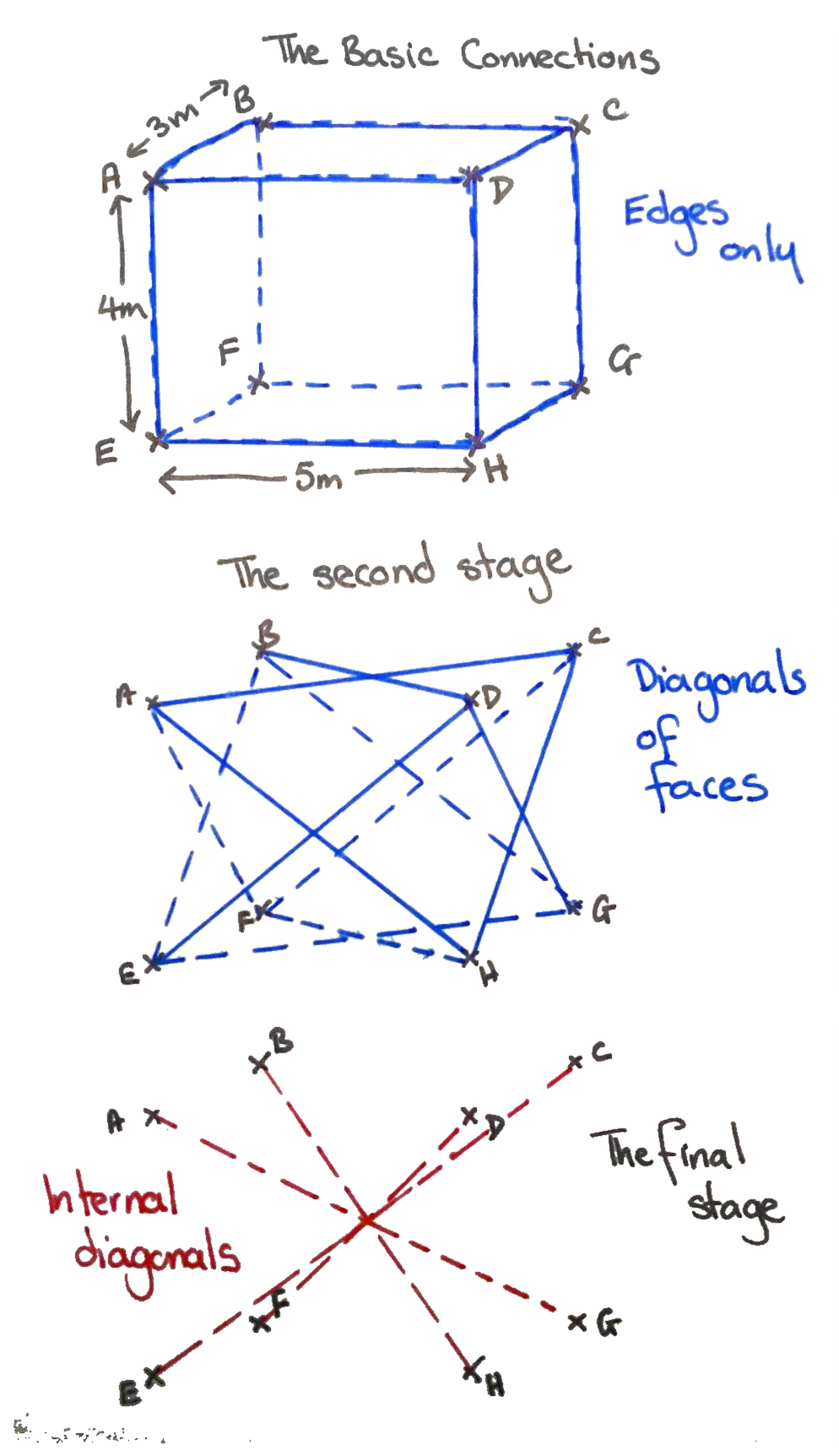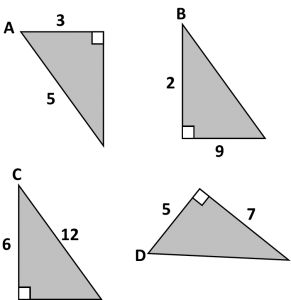# 268. Monkeying with Pythagoras

My (not so little) monkeys in KS3 have been discovering and using Pythagoras’ Theorem. They usually deal with open questions quite well, however this one took a fair bit of discussion. This challenge requires no worksheets or fancy resources, just write it on the board. The context is modified, but the essential question remains the same.

Challenge

Zookeepers have attached eight bolts in a cuboid formation (sides 3m, 4m and 5m) to the trees in a chimpanzee enclosure. The keepers attach taut ropes between the bolts for the chimps to climb on. Each length of rope is individually cut. No length is lost in knots.

• What is the maximum length of any one piece of rope?
• What is the total amount used, if every corner is joined without duplication?

Solution

The first step to solving it is a good diagram of the problem. Students then need to break it down into triangles. The solution has several levels of difficulty:

• Total of the edges of the cuboid
• Total of the diagonals on the faces
• Total of the diagonals across the inside of the cuboid

This diagram demonstrates the levels of the problem – have fun!# 237. Quick Starter

Don’t you just hate it when students forget basic key skills? Especially those at the higher end of Year 11 or studying A-Level, who should have a better core knowledge. What if there was a magic tool which began to address this issue?

Skills required

• Comparing fractions
• Trigonometric ratios
• Simplifying surds
• Rationalising surds
• Pythagoras

Equipment

You will not need:

• Worksheet
• Powerpoint
• Printer
• Laminator
• Calculator

Magic Tool

• One board, with pen

Activity

Quite simply draw the four diagrams below on the board and ask the following questions:1. Which has the largest sine ratio: A or B?
2. Which has the largest cosine ratio: C or D?
3. Which has the smallest tangent ratio: A, B, C or D?
4. Extension: Calculate the missing angles and areas (Calculator allowed)

It takes moments to draw the questions on the board, but the discussion can take some time and addresses several basic skills. You can change the numbers to adjust the level of challenge.

# 215. Another Takeaway

After the warm response the first takeaway homework received, I’ve written another! This time it is about Pythagoras and basic trigonometry – suitable for introductory or revision homework. This one hasn’t yet been trialled, so let me know how it goes.

Pythagoras and Trigonometry homework

# 213. Crack the code 2

If you liked the previous post 212. Crack the code 1, you’ll like this one.

Another Code sheet, but this time on Pythagoras’ theorem. The sheet covers Pythagoras (find hypotenuse and shorter side), Pythagorean triples, problem solving and rounding to 2dp.

# 178. Santa’s Secret

Santa’s secret is that he can get your class to revise harder topics – without them realising!

Equipment

Activity
Paper chains are made from equally sized strips of paper. Each loop is made from a strip of paper, which has one end glued to the other.

Question 1
How many 3cm by 18cm strips of paper can you cut from a sheet of A4 paper? Remember, each strip is made from one complete piece of paper.

Question 2
If each strip has an overlap of 1cm, what is the circumference of the loop made? What is the diameter?

Question 3
When two loops are attached there is an overlap of 0.5cm. How long would a chain of 12 loops be?
Hint: two loops with diameter 4cm would have a combined length of (4+4-0.5)cm = 7.5cm.

Question 4
A room has dimensions 5m by 7m. How far is it diagonally across the room?

Question 5
How many loops would a paper chain have if it reached diagonally across the room?

Extension
To make the chain hang in U shape, rather than stretching flat across the ceiling, 5 extra loops are added per whole metre of chain. How long would the chain diagonally across the room be? How many loops?

Challenge
How many sheets of paper would be required to make enough paper chain to hang in a U shape joining every corner of the room?

# 36. Pythagoras Squirrel

I stumbled across this website ages ago and I still go back for this activity.

Larry the Squirrel

Who knew a crazy squirrel could take the fear out of basic pythagoras?The picture is Secret Squirrel and Morroco Mole – if you remember them, you’ve probably been teaching quite a while …